# Mean State

Period Mean (original grids) [Watt m-2]
Model Period Mean (intersection) [Watt m-2]
Model Period Mean (complement) [Watt m-2]
Benchmark Period Mean (intersection) [Watt m-2]
Benchmark Period Mean (complement) [Watt m-2]
Bias [Watt m-2]
RMSE [Watt m-2]
Phase Shift [months]
Bias Score 
RMSE Score 
Seasonal Cycle Score 
Spatial Distribution Score 
Interannual Variability Score 
Overall Score 
Benchmark [-] 397.
CLM5PHSOFF [-] 396. 396. 396. 408. -0.219 6.93 0.212 0.821 0.497 0.974 0.999 0.754 0.757
CLM5PHSON [-] 395. 395. 396. 408. -0.219 6.93 0.212 0.821 0.497 0.974 0.999 0.754 0.757
Period Mean (original grids) [Watt m-2]
Model Period Mean (intersection) [Watt m-2]
Model Period Mean (complement) [Watt m-2]
Benchmark Period Mean (intersection) [Watt m-2]
Benchmark Period Mean (complement) [Watt m-2]
Bias [Watt m-2]
RMSE [Watt m-2]
Phase Shift [months]
Bias Score 
RMSE Score 
Seasonal Cycle Score 
Spatial Distribution Score 
Interannual Variability Score 
Overall Score 
Benchmark [-] 384.
CLM5PHSOFF [-] 384. 384. 384. 397. -0.0965 9.48 0.181 0.904 0.688 0.985 0.999 0.910 0.862
CLM5PHSON [-] 384. 384. 384. 397. -0.0965 9.48 0.181 0.904 0.688 0.985 0.999 0.910 0.862
Period Mean (original grids) [Watt m-2]
Model Period Mean (intersection) [Watt m-2]
Model Period Mean (complement) [Watt m-2]
Benchmark Period Mean (intersection) [Watt m-2]
Benchmark Period Mean (complement) [Watt m-2]
Bias [Watt m-2]
RMSE [Watt m-2]
Phase Shift [months]
Bias Score 
RMSE Score 
Seasonal Cycle Score 
Spatial Distribution Score 
Interannual Variability Score 
Overall Score 
Benchmark [-] 362.
CLM5PHSOFF [-] 361. 361. 361. 382. 0.0495 8.96 0.237 0.879 0.702 0.971 1.00 0.852 0.851
CLM5PHSON [-] 359. 359. 361. 382. 0.0495 8.96 0.237 0.879 0.702 0.971 1.00 0.852 0.851
Period Mean (original grids) [Watt m-2]
Model Period Mean (intersection) [Watt m-2]
Model Period Mean (complement) [Watt m-2]
Benchmark Period Mean (intersection) [Watt m-2]
Benchmark Period Mean (complement) [Watt m-2]
Bias [Watt m-2]
RMSE [Watt m-2]
Phase Shift [months]
Bias Score 
RMSE Score 
Seasonal Cycle Score 
Spatial Distribution Score 
Interannual Variability Score 
Overall Score 
Benchmark [-] 239.
CLM5PHSOFF [-] 238. 238. 238. 251. 0.254 9.46 0.0140 0.964 0.834 0.999 1.00 0.887 0.920
CLM5PHSON [-] 236. 236. 238. 251. 0.254 9.46 0.0140 0.964 0.834 0.999 1.00 0.887 0.920
Period Mean (original grids) [Watt m-2]
Model Period Mean (intersection) [Watt m-2]
Model Period Mean (complement) [Watt m-2]
Benchmark Period Mean (intersection) [Watt m-2]
Benchmark Period Mean (complement) [Watt m-2]
Bias [Watt m-2]
RMSE [Watt m-2]
Phase Shift [months]
Bias Score 
RMSE Score 
Seasonal Cycle Score 
Spatial Distribution Score 
Interannual Variability Score 
Overall Score 
Benchmark [-] 411.
CLM5PHSOFF [-] 410. 410. 410. 415. 0.0344 5.44 0.531 0.668 0.397 0.922 0.993 0.736 0.686
CLM5PHSON [-] 408. 408. 410. 415. 0.0344 5.44 0.531 0.668 0.397 0.922 0.993 0.736 0.686
Period Mean (original grids) [Watt m-2]
Model Period Mean (intersection) [Watt m-2]
Model Period Mean (complement) [Watt m-2]
Benchmark Period Mean (intersection) [Watt m-2]
Benchmark Period Mean (complement) [Watt m-2]
Bias [Watt m-2]
RMSE [Watt m-2]
Phase Shift [months]
Bias Score 
RMSE Score 
Seasonal Cycle Score 
Spatial Distribution Score 
Interannual Variability Score 
Overall Score 
Benchmark [-] 264.
CLM5PHSOFF [-] 264. 264. 264. 277. 0.0194 8.45 0.00704 0.975 0.861 1.00 1.00 0.811 0.918
CLM5PHSON [-] 264. 263. 264. 277. 0.0194 8.45 0.00704 0.975 0.861 1.00 1.00 0.811 0.918
Period Mean (original grids) [Watt m-2]
Model Period Mean (intersection) [Watt m-2]
Model Period Mean (complement) [Watt m-2]
Benchmark Period Mean (intersection) [Watt m-2]
Benchmark Period Mean (complement) [Watt m-2]
Bias [Watt m-2]
RMSE [Watt m-2]
Phase Shift [months]
Bias Score 
RMSE Score 
Seasonal Cycle Score 
Spatial Distribution Score 
Interannual Variability Score 
Overall Score 
Benchmark [-] 294.
CLM5PHSOFF [-] 294. 293. 293. 336. 0.277 9.99 0.0242 0.939 0.825 0.998 1.00 0.784 0.895
CLM5PHSON [-] 291. 291. 293. 336. 0.277 9.99 0.0242 0.939 0.825 0.998 1.00 0.784 0.895
Period Mean (original grids) [Watt m-2]
Model Period Mean (intersection) [Watt m-2]
Model Period Mean (complement) [Watt m-2]
Benchmark Period Mean (intersection) [Watt m-2]
Benchmark Period Mean (complement) [Watt m-2]
Bias [Watt m-2]
RMSE [Watt m-2]
Phase Shift [months]
Bias Score 
RMSE Score 
Seasonal Cycle Score 
Spatial Distribution Score 
Interannual Variability Score 
Overall Score 
Benchmark [-] 379.
CLM5PHSOFF [-] 376. 377. 377. 410. -0.454 9.29 0.100 0.882 0.746 0.991 1.00 0.877 0.874
CLM5PHSON [-] 371. 372. 377. 410. -0.454 9.29 0.100 0.882 0.746 0.991 1.00 0.877 0.874
Period Mean (original grids) [Watt m-2]
Model Period Mean (intersection) [Watt m-2]
Model Period Mean (complement) [Watt m-2]
Benchmark Period Mean (intersection) [Watt m-2]
Benchmark Period Mean (complement) [Watt m-2]
Bias [Watt m-2]
RMSE [Watt m-2]
Phase Shift [months]
Bias Score 
RMSE Score 
Seasonal Cycle Score 
Spatial Distribution Score 
Interannual Variability Score 
Overall Score 
Benchmark [-] 244.
CLM5PHSOFF [-] 243. 244. 244. 248. 0.0310 8.41 0.00100 0.978 0.842 1.00 1.00 0.883 0.924
CLM5PHSON [-] 243. 243. 244. 248. 0.0310 8.41 0.00100 0.978 0.842 1.00 1.00 0.883 0.924
Period Mean (original grids) [Watt m-2]
Model Period Mean (intersection) [Watt m-2]
Model Period Mean (complement) [Watt m-2]
Benchmark Period Mean (intersection) [Watt m-2]
Benchmark Period Mean (complement) [Watt m-2]
Bias [Watt m-2]
RMSE [Watt m-2]
Phase Shift [months]
Bias Score 
RMSE Score 
Seasonal Cycle Score 
Spatial Distribution Score 
Interannual Variability Score 
Overall Score 
Benchmark [-] 351.
CLM5PHSOFF [-] 350. 350. 350. 359. 0.0571 7.85 0.104 0.931 0.779 0.993 1.00 0.909 0.899
CLM5PHSON [-] 350. 350. 350. 359. 0.0571 7.85 0.104 0.931 0.779 0.993 1.00 0.909 0.899
Period Mean (original grids) [Watt m-2]
Model Period Mean (intersection) [Watt m-2]
Model Period Mean (complement) [Watt m-2]
Benchmark Period Mean (intersection) [Watt m-2]
Benchmark Period Mean (complement) [Watt m-2]
Bias [Watt m-2]
RMSE [Watt m-2]
Phase Shift [months]
Bias Score 
RMSE Score 
Seasonal Cycle Score 
Spatial Distribution Score 
Interannual Variability Score 
Overall Score 
Benchmark [-] 308.
CLM5PHSOFF [-] 307. 307. 307. 324. 0.0546 8.06 0.0712 0.930 0.782 0.995 1.00 0.739 0.871
CLM5PHSON [-] 304. 304. 307. 324. 0.0546 8.06 0.0712 0.930 0.782 0.995 1.00 0.739 0.871
Period Mean (original grids) [Watt m-2]
Model Period Mean (intersection) [Watt m-2]
Model Period Mean (complement) [Watt m-2]
Benchmark Period Mean (intersection) [Watt m-2]
Benchmark Period Mean (complement) [Watt m-2]
Bias [Watt m-2]
RMSE [Watt m-2]
Phase Shift [months]
Bias Score 
RMSE Score 
Seasonal Cycle Score 
Spatial Distribution Score 
Interannual Variability Score 
Overall Score 
Benchmark [-] 344.
CLM5PHSOFF [-] 311. 311. 311. 360. -0.0963 9.16 0.113 0.894 0.716 0.987 1.00 0.838 0.858
CLM5PHSON [-] 308. 308. 311. 360. -0.0963 9.16 0.113 0.894 0.716 0.987 1.00 0.838 0.858
Period Mean (original grids) [Watt m-2]
Model Period Mean (intersection) [Watt m-2]
Model Period Mean (complement) [Watt m-2]
Benchmark Period Mean (intersection) [Watt m-2]
Benchmark Period Mean (complement) [Watt m-2]
Bias [Watt m-2]
RMSE [Watt m-2]
Phase Shift [months]
Bias Score 
RMSE Score 
Seasonal Cycle Score 
Spatial Distribution Score 
Interannual Variability Score 
Overall Score 
Benchmark [-] 343.
CLM5PHSOFF [-] 343. 343. 343. 360. 0.000187 11.3 0.0949 0.917 0.723 0.990 1.00 0.894 0.874
CLM5PHSON [-] 342. 342. 343. 360. 0.000187 11.3 0.0949 0.917 0.723 0.990 1.00 0.894 0.874
Period Mean (original grids) [Watt m-2]
Model Period Mean (intersection) [Watt m-2]
Model Period Mean (complement) [Watt m-2]
Benchmark Period Mean (intersection) [Watt m-2]
Benchmark Period Mean (complement) [Watt m-2]
Bias [Watt m-2]
RMSE [Watt m-2]
Phase Shift [months]
Bias Score 
RMSE Score 
Seasonal Cycle Score 
Spatial Distribution Score 
Interannual Variability Score 
Overall Score 
Benchmark [-] 244.
CLM5PHSOFF [-] 243. 243. 243. 253. -0.0103 8.60 0.0247 0.965 0.833 0.998 1.00 0.881 0.919
CLM5PHSON [-] 243. 243. 243. 253. -0.0103 8.60 0.0247 0.965 0.833 0.998 1.00 0.881 0.919
Period Mean (original grids) [Watt m-2]
Model Period Mean (intersection) [Watt m-2]
Model Period Mean (complement) [Watt m-2]
Benchmark Period Mean (intersection) [Watt m-2]
Benchmark Period Mean (complement) [Watt m-2]
Bias [Watt m-2]
RMSE [Watt m-2]
Phase Shift [months]
Bias Score 
RMSE Score 
Seasonal Cycle Score 
Spatial Distribution Score 
Interannual Variability Score 
Overall Score 
Benchmark [-] 374.
CLM5PHSOFF [-] 369. 369. 369. 398. 0.0604 9.12 0.254 0.816 0.684 0.968 1.00 0.867 0.837
CLM5PHSON [-] 362. 362. 369. 398. 0.0604 9.12 0.254 0.816 0.684 0.968 1.00 0.867 0.837
Period Mean (original grids) [Watt m-2]
Model Period Mean (intersection) [Watt m-2]
Model Period Mean (complement) [Watt m-2]
Benchmark Period Mean (intersection) [Watt m-2]
Benchmark Period Mean (complement) [Watt m-2]
Bias [Watt m-2]
RMSE [Watt m-2]
Phase Shift [months]
Bias Score 
RMSE Score 
Seasonal Cycle Score 
Spatial Distribution Score 
Interannual Variability Score 
Overall Score 
Benchmark [-] 332.
CLM5PHSOFF [-] 331. 331. 331. 370. -0.0972 7.51 0.0275 0.951 0.841 0.998 1.00 0.841 0.912
CLM5PHSON [-] 328. 328. 331. 370. -0.0972 7.51 0.0275 0.951 0.841 0.998 1.00 0.841 0.912
Period Mean (original grids) [Watt m-2]
Model Period Mean (intersection) [Watt m-2]
Model Period Mean (complement) [Watt m-2]
Benchmark Period Mean (intersection) [Watt m-2]
Benchmark Period Mean (complement) [Watt m-2]
Bias [Watt m-2]
RMSE [Watt m-2]
Phase Shift [months]
Bias Score 
RMSE Score 
Seasonal Cycle Score 
Spatial Distribution Score 
Interannual Variability Score 
Overall Score 
Benchmark [-] 277.
CLM5PHSOFF [-] 276. 276. 276. 298. -0.0815 7.66 0.0260 0.950 0.841 0.998 1.00 0.828 0.910
CLM5PHSON [-] 274. 275. 276. 298. -0.0815 7.66 0.0260 0.950 0.841 0.998 1.00 0.828 0.910
Period Mean (original grids) [Watt m-2]
Model Period Mean (intersection) [Watt m-2]
Model Period Mean (complement) [Watt m-2]
Benchmark Period Mean (intersection) [Watt m-2]
Benchmark Period Mean (complement) [Watt m-2]
Bias [Watt m-2]
RMSE [Watt m-2]
Phase Shift [months]
Bias Score 
RMSE Score 
Seasonal Cycle Score 
Spatial Distribution Score 
Interannual Variability Score 
Overall Score 
Benchmark [-] 296.
CLM5PHSOFF [-] 294. 295. 295. 324. -0.178 9.51 0.0536 0.911 0.770 0.996 1.00 0.791 0.873
CLM5PHSON [-] 293. 294. 295. 324. -0.178 9.51 0.0536 0.911 0.770 0.996 1.00 0.791 0.873
Period Mean (original grids) [Watt m-2]
Model Period Mean (intersection) [Watt m-2]
Model Period Mean (complement) [Watt m-2]
Benchmark Period Mean (intersection) [Watt m-2]
Benchmark Period Mean (complement) [Watt m-2]
Bias [Watt m-2]
RMSE [Watt m-2]
Phase Shift [months]
Bias Score 
RMSE Score 
Seasonal Cycle Score 
Spatial Distribution Score 
Interannual Variability Score 
Overall Score 
Benchmark [-] 406.
CLM5PHSOFF [-] 405. 405. 405. 410. -0.270 6.26 0.342 0.796 0.499 0.953 1.00 0.824 0.762
CLM5PHSON [-] 405. 405. 405. 410. -0.270 6.26 0.342 0.796 0.499 0.953 1.00 0.824 0.762
Period Mean (original grids) [Watt m-2]
Model Period Mean (intersection) [Watt m-2]
Model Period Mean (complement) [Watt m-2]
Benchmark Period Mean (intersection) [Watt m-2]
Benchmark Period Mean (complement) [Watt m-2]
Bias [Watt m-2]
RMSE [Watt m-2]
Phase Shift [months]
Bias Score 
RMSE Score 
Seasonal Cycle Score 
Spatial Distribution Score 
Interannual Variability Score 
Overall Score 
Benchmark [-] 376.
CLM5PHSOFF [-] 376. 375. 376. 374. -0.175 9.06 0.144 0.889 0.661 0.985 1.00 0.837 0.839
CLM5PHSON [-] 376. 375. 376. 374. -0.175 9.06 0.144 0.889 0.661 0.985 1.00 0.837 0.839
Period Mean (original grids) [Watt m-2]
Model Period Mean (intersection) [Watt m-2]
Model Period Mean (complement) [Watt m-2]
Benchmark Period Mean (intersection) [Watt m-2]
Benchmark Period Mean (complement) [Watt m-2]
Bias [Watt m-2]
RMSE [Watt m-2]
Phase Shift [months]
Bias Score 
RMSE Score 
Seasonal Cycle Score 
Spatial Distribution Score 
Interannual Variability Score 
Overall Score 
Benchmark [-] 307.
CLM5PHSOFF [-] 305. 304. 303. 340. 1.13 13.5 0.185 0.721 0.579 0.981 0.998 0.747 0.768
CLM5PHSON [-] 301. 301. 303. 340. 1.13 13.5 0.185 0.721 0.579 0.981 0.998 0.747 0.768

# Temporally integrated period mean

BENCHMARK MEAN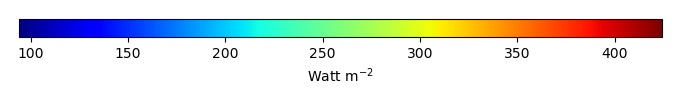MODEL MEANBIAS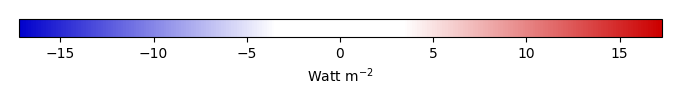BIAS SCORERMSE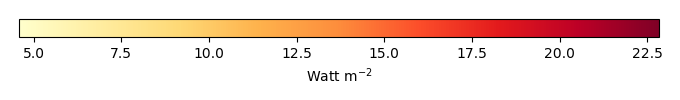RMSE SCOREBENCHMARK INTERANNUAL VARIABILITY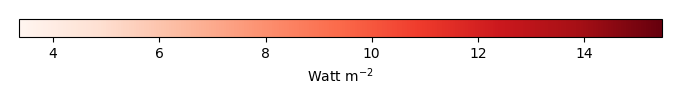MODEL INTERANNUAL VARIABILITYINTERANNUAL VARIABILITY SCOREBENCHMARK MAX MONTHMODEL MAX MONTHDIFFERENCE IN MAX MONTH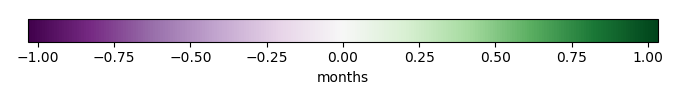SEASONAL CYCLE SCORESPATIAL TAYLOR DIAGRAMMODEL COLORS# Spatially integrated regional mean

MODEL COLORSREGIONAL MEANANNUAL CYCLEMONTHLY ANOMALYANNUAL CYCLE# All Models

BenchmarkCLM5PHSOFFCLM5PHSON# Data Information

creation_date: Mon Jun 30 23:41:07 PDT 2014

Conventions: Please contact Prof. James Randerson (Email: jranders@uci.edu) or Dr. Mingquan Mu (mmu@uci.edu) for any question

source_file: This product is generated from monthly 1 degree GEWEX SRB Radiation observations

title: derived GEWEX SRB Radiation

Approach: I simply read surface all-sky downward longwave (lw_sfc_dn) radiation. Meanwhile, the data were also binned from original 1.0x1.0 resolution to final 0.5x0.5 resolution.

Temporal resolution: monthly

General information: This product was derived from NASA GEWEX SRB radiation dataset.

Spatial resolution: 0.5x0.5 degree